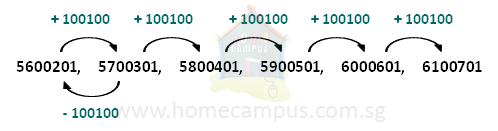## Number Comparison, Ordering and Patterns

Practice Unlimited Questions

#### Rules of Number Comparison

Rule 1: If the two numbers to be compared have different number of digits then the one that has got more digits is greater than the one that has got fewer digits.

Rule 2: If the two numbers have an equal number of digits then you compare the two numbers digit by digit starting from the digits in the highest place value.

#### 1. Is 5647390 less than or greater than 873974?

Number 1: 5647390 (7 digits)
Number 2: 873974 (6 digits)

Since 5647390 has more digits than 873974, therefore,

5647390 is greater than 873974     (5647390  >  873974), or

873974 is less than 5647390     (873974  <  5647390)

#### 2. Is 7854912 less than or greater than 7832109?

Number 1: 7854912 (7 digits)
Number 2: 7832109 (7 digits)

Since both the numbers have an equal number of digits, we compare the values of the two numbers starting from the digits in the highest place.

The digit in the highest place for both the numbers is 7, which means both numbers start with 7 million, so you can't compare them at this point. So, move on to the second highest digit.

The digit in the second highest place for both the numbers is 8, which means both numbers have 8 hundred thousand, so you can't compare them at this point either. So, move on to the third highest digit.

In number 1, this is 5 while in number 2 it is 3. Clearly 5 is greater than 3, which means number 1 has fifty thousand while number 2 has only thirty thousand. The values of the rest of the digits don't matter and we can conclude that number 1 is greater than number 2. So,

7854912 is greater than 7832109     (7854912  >  7832109), or

7832109 is less than 7854912     (7832109  <  7854912)

#### 3. Arrange the following numbers in ascending order and descending order.        653981        674190        523716        23989

Number 1: 653981 (6 digits)
Number 2: 674190 (6 digits)
Number 3: 523716 (6 digits)
Number 4: 23989 (5 digits)

23989 is the smallest number.       ......(Rule 1)

Next, we compare the other 3 numbers, digit by digit, starting from the highest place (hundred thousands) and going right.       ......(Rule 2)

523716 is the second smallest number.

653981 is the third smallest number.

674190 is the biggest of the four numbers.

In ascending order (smallest to largest), the numbers can be arranged as:
23989,    523716,    653981,    674190

In descending order (largest to smallest), the numbers can be arranged as:
674190,    653981,   523716,    23989

#### 4. What are the missing numbers A, B and C below?        ____A____ ,   5700301 ,   5800401 ,   ____B____ ,   ____C____ ,   6100701

Study the given numbers.

5800401  −  5700301  =  100100

So, the rule seems to be - add 100100 to get the next number to the right.

To find the missing number B, add 100100 to 5800401.
B  =  5800401  +  100100  =  5900501

To find the missing number C, add 100100 to B, i.e. 5900501.
C  =  5900501  +  100100  =  6000601

To check if we got the pattern right, add 100100 to C and make sure that it is equal to 6100701.

600601  +  100100  =  6100701

Yes, we got the pattern right!

Finally, to find A, we must subtract 100100 from 5700301.
A  =  5700301  −  100100  =  5600201

The series with all the missing numbers filled in is: